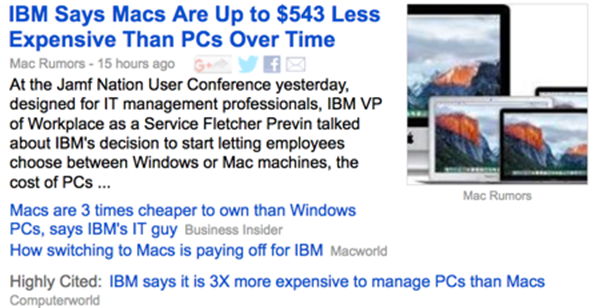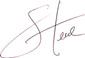## Confusing Expressions of Relative Proportions

During elementary school we learn to reason quantitatively in fundamental ways. One of the concepts that we learn along the way is that of proportions. We are taught to express a value that is greater than another either in terms of multiplication (e.g., “The value of A is three times the value of B”), as a ratio (e.g., a 3 to 1 ratio), as a fraction in which the numerator is greater than the denominator, usually with a denominator of 1  (e.g., 3/1), or as a percentage that is greater than 100% (e.g., 300%). We are taught to express a value that is less than another either as a ratio (e.g., 1 to 3), as a fraction with a numerator that is less than the denominator, usually with a numerator of 1 (e.g., 1/3), or as a percentage that is less than 100% (e.g., 33%). In later years, however, some of us begin to express proportions in confusing and sometimes inaccurate ways.

Consider a case in which the value of A is \$100 and the value of B is \$300. To express the greater value of B proportionally as a percentage of A’s value, would it be accurate to say that B is 300% greater than A? No, it wouldn’t. B is only 200% greater than A (300% – 100% = 200%). It is correct, however, to say that “the value of B is 300% the value of A.” To avoid confusion for most audiences, it usually works better to express this proportional difference in terms of multiplication, such as “The value of B is three times the value of A.”

Confusion can also occur when we describe lesser proportions. Recently, while reading a book by a neuroscientist who has closely studied how humans reason quantitatively, I came across the unexpectedly confusing expression “a million times less.” As I understand it, you can reduce a value through multiplication only by multiplying it by a value that is less than one (e.g., a fraction such as 1/3 or a negative value such as -1). The author should have expressed the lesser proportion as “one millionth,” which is conceptually clear.

Consider the following results that encountered in Google News:Notice the sentence attributed to Business Insider: “Macs are 3 times cheaper to own than Windows PCs…” Is the meaning of this proportion clear? It isn’t clear to me. It makes sense to say that something is three times greater, but not three times less. What the writer should have said was “Macs are one-third as expensive to own as Windows PCs,” or could have reversed the comparison by describing the greater proportion, as Computerworld did: “IBM says it is 3X more expensive to manage PCs than Macs.” When you describe a lesser proportion, express the difference either as a fraction with a numerator that is less than the denominator, usually with a numerator of 1 (e.g., 1/3rd the cost), as a percentage less than 100% (e.g., 33% of the cost), or as a ratio that begins with the smaller value (e.g., a cost ratio of 1 to 3).

People often struggle to understand proportions. We can prevent many of these misunderstandings by expressing proportions properly.

Take care,### 4 Comments on “Confusing Expressions of Relative Proportions”

By Chris. July 17th, 2017 at 4:03 pm

My colleagues are 500% less likely to enjoy the message of this post as I am. :)

Jokes aside, nice article. I have often confused myself with proportions and how to express them, and this helps a lot.

By Stephen Few. July 17th, 2017 at 4:15 pm

Chris,

Actually, “500% less likely” is another great example of this problem. Similar to the equivalent expression “five times less likely,” this percentage-based expression is also confusing–perhaps more so. I suspect that most people are even less inclined to interpret “500% less likely” as “1/5th as likely,” compared to the expression “five times less likely.”

By Andrew. July 18th, 2017 at 8:10 am

Would “500% less likely” be 400% unlikely?